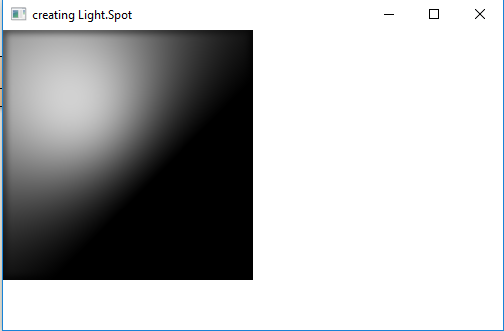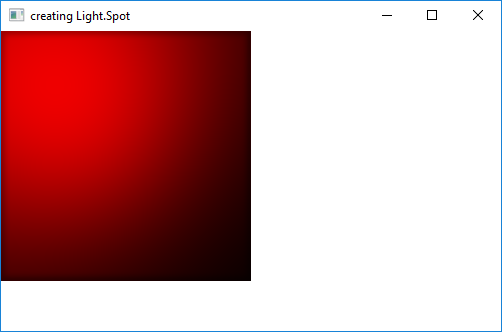# JavaFX | Light.Spot Class

• Last Updated : 21 May, 2019

Light.Spot class is a part of JavaFX. Light.Spot class is used to create a spot light with configurable values of direction vector of light, focus and Color in 3D space. Light.Spot class inherits Light.Point class.

Constructors of the class:

Attention reader! Don’t stop learning now. Get hold of all the important Java Foundation and Collections concepts with the Fundamentals of Java and Java Collections Course at a student-friendly price and become industry ready. To complete your preparation from learning a language to DS Algo and many more,  please refer Complete Interview Preparation Course.

1. Spot(): Creates a spot light with default values
2. Spot(double x, double y, double z, double s, Color c): Creates a spot light with specified values of x, y, z, specularExponent and color of light

Commonly Used Methods:

MethodsExplanation
getPointsAtX()Returns the x coordinate of the direction vector of light.
getPointsAtY()Returns the y coordinate of the direction vector of light.
getPointsAtZ()Returns the z coordinate of the direction vector of light.
getSpecularExponent()Returns the value of specularExponent.
setPointsAtX(double v)Sets the value of x coordinate of the direction vector of light.
setPointsAtY(double v)Sets the value of y coordinate of the direction vector of light.
setPointsAtZ(double v)Sets the value of z coordinate of the direction vector of light.
setSpecularExponent(double v)Sets the value of specularExponent.

Below programs illustrate the use of Light.Spot class:

1. Java Program to create a Spot light and add it to a rectangle: In this program we will create a Rectangle named rectangle with specified height and width. We will also create a Light.Spot object named light. We will set the x, y, z values using setX(), setY() and setZ() function. Now create a lighting object and add the light object to lighting using setLight() function. We will set the Lighting effect to the Rectangle and add it to the scene and add the scene to the stage and call the show function to display the results.
 `// Java Program to create a Spot light``// and add it to a rectangle``import` `javafx.application.Application;``import` `javafx.scene.Scene;``import` `javafx.scene.shape.Rectangle;``import` `javafx.scene.control.*;``import` `javafx.stage.Stage;``import` `javafx.scene.Group;``import` `javafx.scene.effect.Light.*;``import` `javafx.scene.effect.*;``import` `javafx.scene.paint.Color;`` ` `public` `class` `Spot_1 ``extends` `Application {`` ` `    ``// launch the application``    ``public` `void` `start(Stage stage)``    ``{`` ` `        ``// set title for the stage``        ``stage.setTitle(``"creating Light.Spot"``);`` ` `        ``// create Spot Light object``        ``Light.Spot light = ``new` `Light.Spot();`` ` `        ``// set coordinates``        ``light.setX(``100``);``        ``light.setY(``100``);``        ``light.setZ(``100``);`` ` `        ``// create a lighting``        ``Lighting lighting = ``new` `Lighting();`` ` `        ``// set Light of lighting``        ``lighting.setLight(light);`` ` `        ``// create a rectangle``        ``Rectangle rect = ``new` `Rectangle(``250``, ``250``);`` ` `        ``// set fill``        ``rect.setFill(Color.WHITE);`` ` `        ``// set effect``        ``rect.setEffect(lighting);`` ` `        ``// create a Group``        ``Group group = ``new` `Group(rect);`` ` `        ``// create a scene``        ``Scene scene = ``new` `Scene(group, ``500``, ``300``);`` ` `        ``// set the scene``        ``stage.setScene(scene);`` ` `        ``stage.show();``    ``}`` ` `    ``// Main Method``    ``public` `static` `void` `main(String args[])``    ``{`` ` `        ``// launch the application``        ``launch(args);``    ``}``}`

Output:2. Java Program to create a Spotlight and add it to a rectangle and set the coordinates of the direction vector of light and color of light: In this program we will create a Rectangle named rectangle with specified height and width. We will also create a Light.Spot object named light. We will set the x, y, z values using setX(), setY() and setZ() function. The coordinate of the direction vector of light is set using setPointsAtX(), setPointsAtY() and setPointsAtX(), and specify the value of color using setColor() function Now create a lighting object and add the light object to lighting using setLight() function. We will set the Lighting effect to the Rectangle and add it to the scene and add the scene to the stage and call the show function to display the results.
 `// Java Program to create a Spot light``// and add it to a rectangle and set the``// coordinates of direction vector of ``// light and color of light``import` `javafx.application.Application;``import` `javafx.scene.Scene;``import` `javafx.scene.shape.Rectangle;``import` `javafx.scene.control.*;``import` `javafx.stage.Stage;``import` `javafx.scene.Group;``import` `javafx.scene.effect.Light.*;``import` `javafx.scene.effect.*;``import` `javafx.scene.paint.Color;`` ` `public` `class` `Spot_2 ``extends` `Application {`` ` `    ``// launch the application``    ``public` `void` `start(Stage stage)``    ``{`` ` `        ``// set title for the stage``        ``stage.setTitle(``"creating Light.Spot"``);`` ` `        ``// create Spot Light object``        ``Light.Spot light = ``new` `Light.Spot();`` ` `        ``// set coordinate of direction``        ``// the vector of this light``        ``light.setPointsAtX(``0``);``        ``light.setPointsAtY(``0``);``        ``light.setPointsAtZ(-``60``);`` ` `        ``// set specular exponent``        ``light.setSpecularExponent(``2``);`` ` `        ``// set color of light``        ``light.setColor(Color.RED);`` ` `        ``// set coordinates``        ``light.setX(``100``);``        ``light.setY(``100``);``        ``light.setZ(``200``);`` ` `        ``// create a lighting``        ``Lighting lighting = ``new` `Lighting();`` ` `        ``// set Light of lighting``        ``lighting.setLight(light);`` ` `        ``// create a rectangle``        ``Rectangle rect = ``new` `Rectangle(``250``, ``250``);`` ` `        ``// set fill``        ``rect.setFill(Color.WHITE);`` ` `        ``// set effect``        ``rect.setEffect(lighting);`` ` `        ``// create a Group``        ``Group group = ``new` `Group(rect);`` ` `        ``// create a scene``        ``Scene scene = ``new` `Scene(group, ``500``, ``300``);`` ` `        ``// set the scene``        ``stage.setScene(scene);`` ` `        ``stage.show();``    ``}`` ` `    ``// Main Method``    ``public` `static` `void` `main(String args[])``    ``{`` ` `        ``// launch the application``        ``launch(args);``    ``}``}`

Output:Note: The above programs might not run in an online IDE. Please use an offline compiler.

My Personal Notes arrow_drop_up